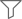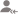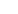true

Find the best tutors and institutes for Vedic Maths Training

Find Best Vedic Maths Training classes

No matching category found.

No matching Locality found.

Outside India?

Search for topicsAll

Lessons

DiscussionLesson Posted on 24/12/2019 Life Skills Coaching/Vedic Maths Training CBSE/Class 8Nikhil Chandra Gayam

I'm studying BE 4th year computer science at Chaitanya Bharathi Institute of Technology(CBIT). My TSEamcet...

Have you felt that squaring a number is the tedious process? Well, not at least for numbers ending with 5. Here I present a simple trick which helps you calculate the squares of 2 digit and 3 digit numbers ending with 5 within seconds. Let's get into the trick!! Let's start with easy number 15 all of... read more

Have you felt that squaring a number is the tedious process? Well, not at least for numbers ending with 5. Here I present a simple trick which helps you calculate the squares of 2 digit and 3 digit numbers ending with 5 within seconds. Let's get into the trick!!

Let's start with easy number 15 all of you know the square of 15 is 225.

Here 15 has two digits which are 1 and 5

There are three simple steps  in this trick

Step 1:

Add 1 to number apart from last digit 5 here it is 1.

1+1=2

Step 2:

Now multiply 1 with the result 2

1*2=2

Step 3:

Now we know 52 =25

Just attach the number obtained in step 2 with 25 which is 225

And it's done!

For clear understanding let's take another example

75

Step 1:

7+1=8

Step 2:

7*8=56

Step 3:

Attach 56 with 25 which is 5625

752 =5625

Very Simple right and you can use this principle for all numbers ending with 5

Let's take a 3 digit number 105

Step 1:

10+1=11

Step 2:

10*11=110

Step 3:

Attach 110 with 25 which is 11025

1052=11025

I hope the concept is clear by now, Ask me if you have doubts.

Happy Learning!

Dislike Bookmark Share

Answered on 08 Jan Life Skills Coaching/Vedic Maths TrainingShubham Tandon

Quantitative Aptitude Mathematical Reasoning Trainer

As the old proverb says "Practice makes a man perfect". Becoming a Master in Mathematics is no different either. However to achieve that a good mentor plays an important role too who can give you guidance in achieving the goal with minimal time and effort. Both Magnitude and Direction are essential.... read more

As the old proverb says "Practice makes a man perfect". Becoming a Master in Mathematics is no different either. However to achieve that a good mentor plays an important role too who can give you guidance in achieving the goal with minimal time and effort. Both Magnitude and Direction are essential.

Dislike Bookmark Share

Lesson Posted on 29/08/2019 Life Skills Coaching/Vedic Maths TrainingRakesh Kumar Sharma

Imparting teaching since 1987-88. Class Room, Online, Workshop, Online, any mode. Written book on Vedic...

9999 x 9999 235 x 235 777 x 777 102x102x102 978x978 Do you know the techniques are lying in logical math? Any question of this sought, If you know, any of them, please reply and get appreciation. And if you don't, let us know we will make it clear. Mathematics is easy. Just enjoy. read more
1. 9999 x 9999
2. 235 x 235
3. 777 x 777
4. 102x102x102
5. 978x978
6. Do you know the techniques are lying in logical math?

Any question of this sought, If you know, any of them, please reply and get appreciation.

And if you don't, let us know we will make it clear.

Mathematics is easy. Just enjoy.

Dislike Bookmark Share

Overview

Questions 34

Lessons 23

Total Shares15,528 Followers

Lesson Posted on 15/08/2019 Life Skills Coaching/Vedic Maths TrainingNamita

I am an experienced, qualified teacher in handwriting and tutor with over 5 years of experience in teaching...

Multiply 2 digit number ended with 5 Example 25*25 Step 1- multiply 5*5 and write it on a paper Step2-multiply 2*2 and then add the same number 2 one times i.e 2*2=4 , 4 + 2 =6 Step 3 - 625 is the answer
Dislike Bookmark Share

Answered on 25/05/2019 Life Skills Coaching/Vedic Maths TrainingParamita

Maths and Science Female Tutor

5000
Dislike Bookmark Share

Lesson Posted on 18/04/2018 CBSE/Class 1/Maths Life Skills Coaching/Vedic Maths Training CBSE/Class 2/Maths/Mental Mathematics +1Ritesh

I will explain a simple and fastest process of multiplication. This can help you to get answers faster. Let's assume you have to multiply 9999 X 2456. Now think how much time it will take? Maybe 30 sec. or 50 sec. or more as well.. Now try this: 9999 x 2456 --------------- 24557544 ... read more

I will explain a simple and fastest process of multiplication. This can help you to get answers faster.

Let's assume you have to multiply 9999 X 2456.

Now think how much time it will take? Maybe 30 sec. or 50 sec. or more as well..

Now try this:

9999

x 2456

---------------

24557544

I didn't use any calculator. let me explain the steps.

Step 1. Subtract 6 (last number) from 10 = 4 (10-6)

Step 2. Rest numbers you can subtract from 9, so (9-5) , (9-4) , (9-2) = 4 , 5 , 7

Step 3. Now subtract 1 from 2456 = 2457

Step 4. You got the answer. i.e 2457 754 4

This is just a small example and this will only work if you have one multiplier with 9's only. I know you won't get always similar but for other, there is a different rule. I hope i will get another quick lesson for that. Or reach me to learn this types of tricks which can make your life easy.

Dislike Bookmark Share

## Top Contributors

Connect with Expert Tutors & Institutes for Vedic Maths Training

Lesson Posted on 19/09/2017 Life Skills Coaching/Vedic Maths Training Tuition/Class I-V Tuition Exam Coaching/Math OlympiadMamta Mund

I have more than 5 years of experience in teaching maths. Now I m working as subject matter expert for...

As we know in decimal system ten digits are used. I.e 0 - 9. Subtracting a number from base 10, 100, 1000. An easy way to calculate is just subtracting all digits of a digit from 9 and last digit by 10.For an example, subtracting 56 from 100: 100 - 56 = (9 - 5) | (10 - 6) = 44Let's check it using... read more

As we know in decimal system ten digits are used. I.e 0 - 9. Subtracting a number from base 10, 100, 1000. An easy way to calculate is just subtracting all digits of a digit from 9 and last digit by 10.

For an example, subtracting 56 from 100:

100 - 56 = (9 - 5) | (10 - 6) = 44

Let's check it using our conventional method, in units place it is 6 there. So carry from tens place, there also 0, so so take carry from hundred places, I.e in units place has value 10 so subtracting 6 from 10 we get 4 and now in tens place there is digit 9. Now subtract 5 from 9 we get 9 - 5 = 4. So our answer is 44. So lengthy using carry.

If we use the first method we have to do just only subtract 9 from all digits and last digit from 10. This is one of the powerful sutra "All From 9 And Last From 10" Of Vedic Mathematics. We also know that complement of 56 is 44. As if we add 56 with 44 we get 100.

Next, try for subtracting 65 from 10000:

10000 - 65 = 10000 - 0065 = 9935.

Dislike Bookmark Share

Lesson Posted on 28/02/2017 Tuition/Class IX-X Tuition Tuition/Class XI-XII Tuition (PUC) Life Skills Coaching/Vedic Maths TrainingNaimish

Vedic Mathematics is a very powerful tool for us to get quick calculations For Multiplication of two 2-digited numbers, we can follow FOIL technique. If you want to multiply 74×36(let's present it as ab×cd) Find 7×3×100=2100 (a×c×100)----------multiply First... read more

Vedic Mathematics is a very powerful tool for us to get quick calculations

For Multiplication of two 2-digited numbers, we can follow FOIL technique.

If you want to multiply 74×36(let's present it as ab×cd)

Find 7×3×100=2100    (a×c×100)----------multiply First to first digits-----F

Find  (4×3+ 7×6)×10=540  [(bc+ad)×10]----------multiply outside and inside digits and multiply their sum with 10-----OI

Find 4×6=24     ( b×d) -------multiply last to last----------L

Add all  2100                                                                                                                                         + 540                                                                                                                                         +   24                                                                                                                                ____________                                                                                                                                     2664

That's the product.

Dislike Bookmark Share

Lesson Posted on 28/02/2017 Tuition/Class IX-X Tuition Tuition/Class XI-XII Tuition (PUC) Life Skills Coaching/Vedic Maths TrainingNaimish

In case of two 2-digited numbers whose digits in 10's place are same and sum of the digits in unit places is 10 For example:- 38 and 32 (10's place digits are equal and 8+2=10) 38×32 can be found very easily 3×(3+1)×100=1200 (the number in 10's place × next number tio it×100 8×2... read more

In case of two 2-digited numbers whose digits in 10's place are same and sum of the digits in unit places is 10

For example:-  38 and 32  (10's place digits are equal and 8+2=10)

38×32 can be found very easily

3×(3+1)×100=1200   (the number in 10's place × next number tio it×100

8×2  =16  (product of digits in unit places) add the result and get the product

38×32=1200+25=1225

That's the result.

Dislike Bookmark Share

## Vedic Maths Training in:

Answered on 24/05/2016 Life Skills Coaching/Vedic Maths TrainingMamta D.

Certified Vedic Maths Trainer

Abacus is limited. Vedic maths is unlimited. Abacus is more about addition subtraction. Whereas Vedic maths trains you in everything like addition subtraction, multiplication division, squares, square root, cube root..so on.. Vedic mathematics can be taught to the kid as small as 8 yrs old..
Dislike Bookmark Share

UrbanPro.com helps you to connect with the best Vedic Maths Training classes in India. Post Your Requirement today and get connected.

x

X

### Looking for Vedic Maths Training Classes?

Find best tutors for Vedic Maths Training Classes by posting a requirement.

• Post a learning requirement
• Get customized responses
• Compare and select the best### Looking for Vedic Maths Training Classes?

Find best Vedic Maths Training Classes in your locality on UrbanProUrbanPro.com is India's largest network of most trusted tutors and institutes. Over 25 lakh students rely on UrbanPro.com, to fulfill their learning requirements across 1,000+ categories. Using UrbanPro.com, parents, and students can compare multiple Tutors and Institutes and choose the one that best suits their requirements. More than 6.5 lakh verified Tutors and Institutes are helping millions of students every day and growing their tutoring business on UrbanPro.com. Whether you are looking for a tutor to learn mathematics, a German language trainer to brush up your German language skills or an institute to upgrade your IT skills, we have got the best selection of Tutors and Training Institutes for you. Read more